# Texas Go Math Grade 4 Lesson 12.4 Answer Key Model Area Formulas

Refer to our Texas Go Math Grade 4 Answer Key Pdf to score good marks in the exams. Test yourself by practicing the problems from Texas Go Math Grade 4 Lesson 12.4 Answer Key Model Area Formulas.

## Texas Go Math Grade 4 Lesson 12.4 Answer Key Model Area Formulas

Essential Question

How can you use a formula to find the area of a rectangle?
Rectangle consists of four sides and the sum of all length of sides is the perimeter of rectangle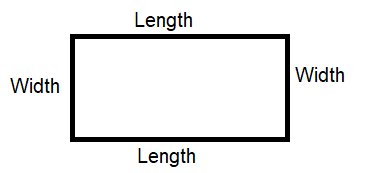Area of a Rectangle = length x width
Remember

Perpendicular lines and perpendicular line segments form right angles.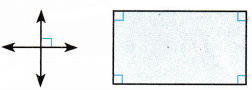Unlock the Problem

The length, l, of a rectangle, can be the measure of any side. The width, w, is the measure of a side perpendicular to the side used as the length.A unit square is a square that is 1 unit long and 1 unit wide. Area is the number of unit squares needed to cover a flat surface without any gaps or overlaps. The area of a unit square is 1 square unit. To find the area of a figure, count the number of unit squares inside the figure. The area is expressed in square units.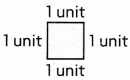How are the length, width, and area of a rectangle related?
The length and width of a rectangles are different,
the length is more or less then width, not same but forms right angle.

Complete the table to find the area.

What relationship do you see among the length, the width, and the area? Write a formula for the area of a rectangle. Use the letter A for area, the letter l for length, and the letter w for width.

Use a Formula You can use a formula to find the area.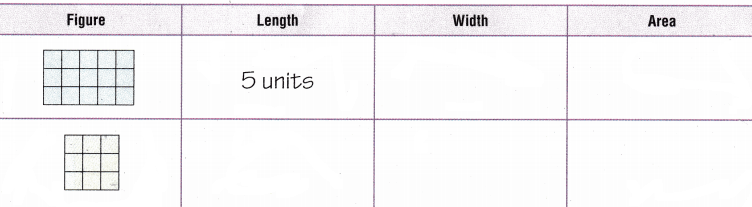Examples Use a formula to find the area of a rectangle and a square.

(A)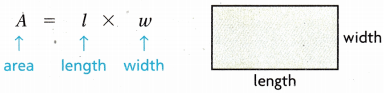A = l × w
= ___________ × ___________ = ___________
The area is ___________ .
A = l × w
= 3 x 5 = 15 square units
The area is 15 square units .

A = l × w
= 3 x 3 = 9 square units
The area is 9 square units .
Explanation:
Rectangle consists of four sides and the sum of all length of sides is the perimeter of rectangle.
A = l × w(B)A = l × w
= ___________ × ___________ = ___________
The area is ___________ .
A = l x w or side x side
=2 x 2
The area is 4 square units.
Explanation:
The area of a square is the amount of surface the shape covers and is measured in square units.
A = l x w or side x side
length = 2; width = 2

Share and Show

Find the area of the rectangle.A = l × ___________
= ___________ × ___________ = ___________
A = l × w
= 11 × 13 = 143 sq cm
Explanation:
A rectangle consists of four sides and the sum of all lengths of sides is the perimeter of a rectangle.
A = l × w
l = length; w = width

Find the area of the rectangle or square.

Question 2.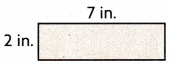14 sq cm
Explanation:
Rectangle consists of four sides and the sum of all length of sides is the perimeter of rectangle.
A = l × w
length = 2 in; width = 7 in
= 7 × 2 = 14 sq cm

Question 3.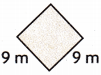81 sq m
Explanation:
The area of a square is the amount of surface the shape covers and is measured in square units.
A = side x side
= 9 × 9 = 81 sq m

Question 4.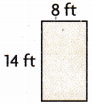112 sqft
Explanation:
Rectangle consists of four sides and the sum of all length of sides is the perimeter of rectangle.
A = l × w
length = 8; width = 14
A = 8 × 14 = 112 sq ft

Practice: Copy and Solve Find the area of the rectangle.

Question 5.
length: 16 feet
width: 6 feet
96 square feet
Explanation:
Area of rectangle = l × w
= 16 × 6
= 96 sq feet

length: 9 yards
width: 17 yards
153 square yards
Explanation:
Area of rectangle = l × w
= 9 × 17
= 153 sq yards

Question 7.
length: 14 centimeters
width: 11 centimeters
154 square cm
Explanation:
Area of rectangle = l × w
= 14 × 11
= 154 sq cm

Problem Solving

Question 8.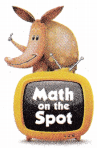H.O.T. Multi-Step Nancy and Luke are drawing plans for rectangular flower gardens. In Nancy’s plan, the garden is 18 feet by 12 feet. In Luke’s plan, the garden is 15 feet by 15 feet. Who drew the garden plan with the greater area? What is the area?
(A) Luke; 205 square feet
(B) Nancy; 206 square feet
(C) Nancy; 216 square feet
(D) Luke; 225 square feet
Option(D)
Explanation: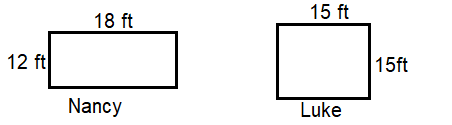Nancy garden area
A = l × w
= 18 × 12 = 218 sq ft
Luke garden area
A = Side × Side
= 15 × 15 = 225 sq ft

a. What do you need to find?
Luke garden area is greater than Nancy garden area.

b. What formula will you use?
Nancy garden area
A = l × w ( rectangle shape, length and width are different)
= 18 × 12 = 218 sq ft
Luke garden area
A = Side × Side (Square shape, length and width are same)
= 15 × 15 = 225 sq ft

c. What units will you use to write the answer?
Square feet

d. Show the steps to solve the problem.
Nancy garden area
A = l × w
= 18 × 12 = 218 sq ft
Luke garden area
A = Side × Side
= 15 × 15 = 225 sq f

e. Complete the sentences.
The area of Nancy’s garden is
_______________________.
The area of Luke’s garden is
_______________________.
___________ garden has the greater area.
The area of Nancy’s garden is 216 sq ft.
The area of Luke’s garden is 225 sq ft.
Luke garden has the greater area.

H.O.T. Use Diagrams Find the area of the rectangle.(A) 32 square feet
(B) 88 square feet
(C) 336 square feet
(D) 384 square feet
Option(D)
Explanation:
Length = 4 x 8 =32 ft
width = 4 x 3 = 12 ft
Area of rectangle = l × w
= 32 x 12
= 384 sq ft

Question 10.
Evaluate Sonia is buying carpet for the dining room, which measures 15 feet by 12 feet. How many square feet of carpet does Sonia need to cover the dining room?
(A) 45 square feet
(B) 54 square feet
(C) 170 square feet
(D) 180 square feet
Option(D)
Explanation:
length = 15 feet
width = 12 feet
A = l × w
= 15 x 12
= 180 sq ft

Question 11.
The shape of Denzel’s bedroom floor is shown below. What is the area of the floor?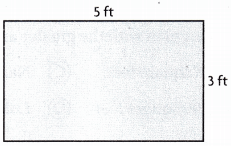(A) 15 square feet
(B) 8 square feet
(C) 2 square feet
(D) 16 square feet
Option(A)
Explanation:
length = 15 ft
width = 15 ft
A = l × w
= 5 x 3 = 15 sq ft

Question 12.
Ms. Jenkins is buying tile for her kitchen floor, which measures 17 feet by 12 feet. How many square feet of tile does Ms. Jenkins need to cover the kitchen floor?
(A) 58 square feet
(B) 194 square feet
(C) 204 square feet

(D) 29 square feet
Option (C)
Explanation:
length = 17 ft
width = 12 ft
A = l × w
= 17 x 12
= 204 sq feet

Area Model Go Math 4th Grade Lesson 12.4 Question 13.
Multi-Step Jasmin’s rectangular backyard is 15 yards by 18 yards. Carolyn’s rectangular backyard is 20 yards by 9 yards. How much greater is the area of Jasmine’s yard than Carolyn’s?
(A) 8 square yards
(B) 4 square yards
(C) 50 square yards
(D) 90 square yards
Option(D)
Explanation:
Jasmin’s rectangular backyard is 15 yards by 18 yards.
A = l x w
15 x 18 = 270 sq yds
Carolyn’s rectangular backyard is 20 yards by 9 yards.
A = l x w
20 x 9 = 180 sq yds
The area of Jasmine’s yard is greater than Carolyn’s area
270 – 180 = 90 sq yds

TEXAS Test Prep

Question 14.
Barry is building a dog house. He wants to put linoleum on the floor. The floor of the dog house is a rectangle that is 56 inches long. The width is half its length. How many square inches of linoleum does Barry need for his dog house?
(A) 6,272 square inches
(B) 336 square inches
(C) 168 square inches
(D) 1,568 square inches
Option(D)
Explanation:
length = 56 inches long.
width is half its length = 56 ÷ 2 = 28 inches
A = l x w
56 x 28 = 1,568 square units

### Texas Go Math Grade 4 Lesson 12.4 Homework and Practice Answer Key

Find the area of the square or rectangle.

Question 1.18 square inches
Explanation:
Area of rectangle = l × w
= 6 x 3 = 18 sq in

Question 2.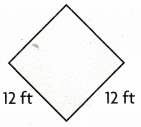144 square feet
Explanation:
Area of square = s × s
= 12 x 12 = 144 sq ft

Question 3.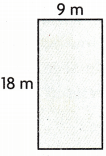162 square meters
Explanation:
Area of rectangle = l × w
= 18 x 9 = 162 sq m

Question 4.240 square yards
Explanation:
Area of rectangle = l × w
= 10 x 24 = 240 sq yd

Find the area of the rectangle.

Question 5.
length: 25 centimeters
width: 10 centimeters
250 square centimeters
Explanation:
Area of rectangle = l × w
= 25 x 10 = 250 sq cm

length: 8 feet
width: 4 feet
32 square feet
Explanation:
Area of rectangle = l × w
= 8 x 4 = 32 sq ft

Question 7.
length: 12 yards
width: 10 yards
120 square yards
Explanation:
Area of rectangle = l x w
= 12 x 10 = 120 sq yards

Question 8.
length: 22 inches
width: 5 inches
110 square inches
Explanation:
Area of rectangle = l × w
=22 x 5 = 110 sq inches

Problem Solving

Question 9.
Joann will cover a bulletin board with paper. The board measures 6 feet by 4 feet. How many square feet of paper does JoAnn need to cover the board?
24 square feet
Explanation:
Area of rectangle = l × w
length = 6; width = 4
= 6 x 4 =  24 sq ft

Question 10.
Frank will paint a wall that measures 10 feet by 14 feet. What is the area of the wall that Frank will paint?
140 square feet
Explanation:
Area of rectangle = l × w
length = 10; width = 14
=10 x 14 = 140 sq ft

Lesson Check

Question 11.
The length of a basketball court is 94 feet. The width of the court is 50 feet. What is the area of the court?
(A) 4,700 sq ft
(B) 288 sq ft
(C) 47o sq ft
(D) 2,880 sq ft
Option(A)
Explanation:
A = l × w
l = 94ft; w = 50ft
=94 x 50 = 4700 sq ft

Question 12.
Carmen sewed a baby quilt that measures 36 inches on each side. What is the area of the quilt?
(A) 1,266 sq in.
(B) 324 sq in.
(C) 144 sq in.
(D) 1296 sq in.
Option(D)
Explanation:
A = s × s
= 36 x 36 = 1296 sq in

Question 13.
Amy bought a new rug for her hallway. The length of the rug is 15 feet and the width of the rug is 3 feet. What is the area of the rug?
(A) 90 sq ft
(B) 45 sq ft
(C) 225 sq ft
(D) 36 sq ft
Option(B)
Explanation:
A = l × w
l = 15ft; w = 3ft
= 15 x 3 = 45 Sq ft

4th Grade Go Math Area Model Lesson 12.4 Question 14.
Mr. Cram wants to decorate his door for the first day of school. The door is 7 feet tall and 3 feet wide. How many square feet of paper will Mr. Cram need to cover the door before he decorates it?
(A) 28 sq ft
(B) 20 sq ft
(C) 21 sq ft
(D) 49 sq ft
option(C)
Explanation:
A = l × w
l = 7ft; w = 3ft
=7 x 3 = 21 sq ft

Question 15.
Multi-Step Xavier wants to buy fertilizer for his yard. The yard measures 35 feet by 55 feet. The directions on the hag of fertilizer say that one bag will cover 1,250 sq ft. How many bags of fertilizer should Xavier buy?
(A) 2
(B) 4
(C) 3
(D) 1
Option(A)
Explanation:
A = l × w
l = 35ft; w = 55ft
= 35 x 55 = 1925 sq ft
One bag covers 1250 sq ft,
Xavier wants 2 bags of fertilizer to cover 1925 sq ft of yard.

Question 16.
Multi-Step Jean will cover the front and back of a folder with craft paper. The folder measures 29 centimeters by 24 centimeters. How many square centimeters of craft paper will Jean use?
(A) 696 sq cm
(B) 212 sq cm
(C) 576 sq cm
(D) 1,392 sq cm